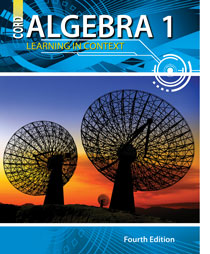Welcome to CORD Online Resources For Learning in Context

Learning in Context is an on-line supplement for our math and science textbooks. Each lesson in CORD’s textbooks is supported by a series of links to various websites. These links add new or more in-depth meaning to the lessons taught in the textbook.

You can navigate each book by using the expanding menu at the left or clicking on the chapters below.

Algebra 1 - 4th EditionChapters

Chapter 1: Fundamentals for Algebra

Chapter 2: Solving Equations

Chapter 3: Inequalities

Chapter 4: Linear Equations

Chapter 5: Functions

Chapter 7: Polynomials and Factors

Chapter 8: Quadratic Equations and Functions

Chapter 10: Rational Expressions and Equations

Chapter 11: Statistics